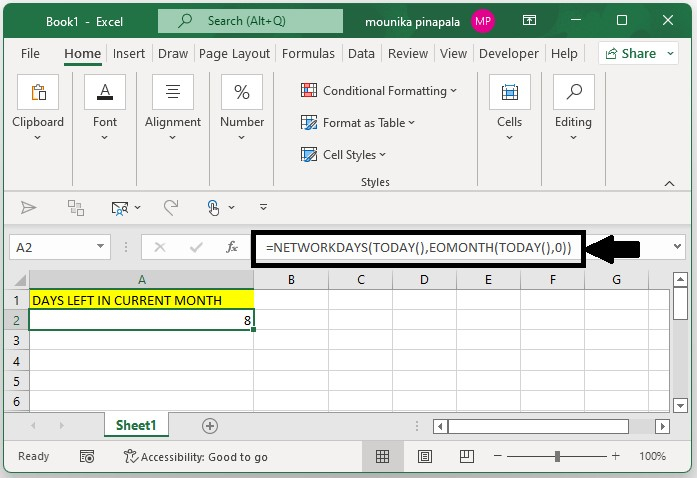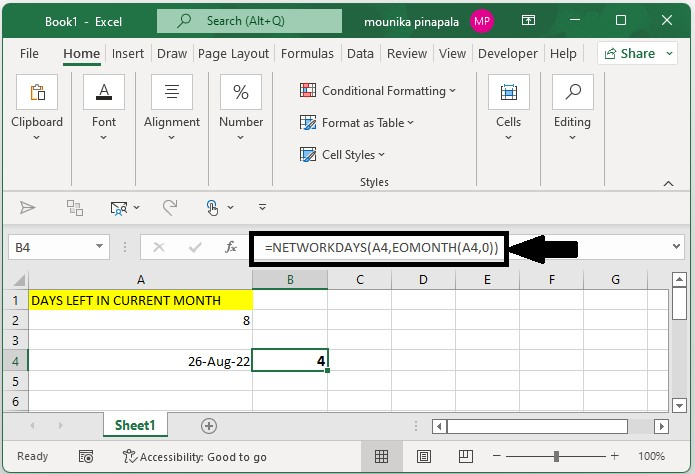# How to calculate the number of working days left in the current month in Excel?

In this tutorial, we will use a simple example to show how you can use Excel to calculate the number of working days left from the current month in a Microsoft Excel sheet using the formulas.

## Step 1

Consider that you want to calculate the number of working days left from today’s date (today’s date is 22 Aug 2022) in the current month using the below formula.

=NETWORKDAYS(TODAY(),EOMONTH(TODAY(),0))

## Step 2

Open a Microsoft excel sheet, you need to enter the above mentioned formula into the formula bar or in a blank cell in the excel sheet and press enter key to get the working days left in the current month. Kindly find the below screenshot for your reference.## Step 2

In the above screenshot, you can see that total of 8 working days is left in this month or in the current month from today.

Note − If you want to calculate the number of working days that are left in the current month by giving a particular or certain date, then you need to apply the below given formula. Kindly find the below given screenshot for your reference.

=NETWORKDAYS(A4,EOMONTH(A4,0))Here, A4 is a specific date that I have given to calculate the working days from the current date to a specific date in the current month.

## Conclusion

This is how you can use the features available in Excel to calculate the number of working days left in the current month and the number of working days left on a specific date from today in a current month.

Updated on: 06-Feb-2023

169 Views